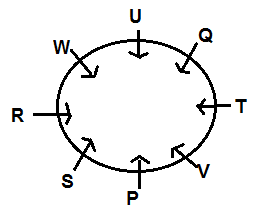Latest Banking jobs   »

# Reasoning Ability Quiz For SBI PO Prelims 2022- 15th December

Directions (1–5): Study the following information carefully and answer the questions given below.
(a) If only conclusion I is true
(b) If only conclusion II is true
(c) If either conclusion I or conclusion II is true
(d) If neither conclusion I nor conclusion II is true
(e) If both conclusions I and II are true

Q1. Statements: H = W, R > F, W ≤ R, F>S,
Conclusions: I. R > H
II. R > S

Q2. Statements: T > K, D ≥ K, M > T
Conclusions: I. D < M
II. M ≥ D

Q3. Statements: R ≤N, F > B, N ≥F
Conclusions: I. N > B
II. B ≤ R

Q4. Statements: H > W, M ≥K, W > M
Conclusions: I. W > K
II. H ≤ M

Q5. Statements: R ≥T, M > D≥Q, T = M,
Conclusions: I. Q < R
II. R ≥ M

Directions (6-10): In these questions, relationship between different elements is shown in the statements. The statements are followed by conclusions.
(a) If only conclusion I is true
(b) If only conclusion II is true
(c) If either conclusion I or II is true
(d) If neither conclusion I nor II is true
(e) If both conclusions I and II are true

Q6. Statements: X ≥ G = H; G > J ≥ L; J ≥ K < Y
Conclusions: I. Y > L
II. K < X

Q7. Statements: A > B = R ≥ S ≥ T; X < J ≤ K < T
Conclusions: I. T > X
II. A > J

Q8. Statements: M > L ≥ K ≤ J; N ≥ R ≥ S = M
Conclusion: I. N > J
II. J ≥ N

Q9. Statements: C ≥ D = E; A = B ≤ S ≥ C
Conclusion: I. E < A
II. B ≤ D

Q10. Statements: X ≥ G > H ≥ I; M > H ≥ L
Conclusion: I. X > L
II. M > I

Directions (11-15): Study the following information carefully to answer the given questions.
There are eight employees i.e., P, Q, R, S, T, U, V and W who have decided to do the lunch in Restaurant. They have seated around a circular table and facing towards the centre but not necessarily in the same order. T sits 2nd right of P.R is not an immediate neighbour of P and T. S is not an immediate neighbour of W. There are two employees between R and Q who sits 2nd left of W. W sits opposite to V who is an immediate neighbour of both P and T.

Q11. Who among the following sits immediate left of V?
(a) P
(b) S
(c) T
(d) U
(e) None of these

Q12. How many employees sit between S and T, clockwise counting from S?
(a) Two
(b) Five
(c) One
(d) Four
(e) No one

Q13. Who among the following sits immediate left of S?
(a) V
(b) U
(c)Q
(d) R
(e) T

Q14. Who among the following sits 2nd to the right of R?
(a) U
(b) P
(c) V
(d) W
(e) None of these

Q15. If Q is related to S and T is related to R, then U is related to which employee?
(a) V
(b) Q
(c) P
(d) W
(e) None of these

Solutions

S1. Ans. (b)
Sol. I. R > H (false)
II. R > S(true)

S2. Ans. (d)
Sol. I. D < M (false)
II. M ≥ D (false)

S3. Ans. (a)
Sol. I. N > B(true)
II. B ≤ R(false)

S4. Ans. (a)
Sol. I. W > K (true)
II. H ≤ M(false)

S5. Ans. (e)
Sol. I. Q < R (true)
II. R ≥ M (true)

S6. Ans. (b)
S7. Ans. (e)
S8. Ans. (c)
S9. Ans. (c)
S10. Ans. (e)

Solutions (11-15):
Sol.S11. Ans. (a)
S12. Ans. (d)
S13. Ans. (d)
S14. Ans. (b)
S15. Ans. (c)#### Congratulations!Union Budget 2023-24: Free PDF